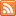# Content Tagged “Random Variable”## Did I Get This – Standardized Scores (z-scores)

Published: Feb 8th, 2013

Scores on the final exam in Professor Meyer’s statistics class follow a normal distribution, with a mean of 82 and a standard deviation of 5. This document is linked from Standard […]

## Learn by Doing – Normal Random Variables

Published: Feb 8th, 2013

The purpose of this interactive activity is to: Get a better feel for the behavior of normal random variables. Check the accuracy of the Standard Deviation Rule. Do some other […]

## Did I Get This – Using the Standard Deviation Rule

Published: Feb 8th, 2013

Length (in days) of human pregnancies is a normal random variable (X) with mean 266, standard deviation 16. For the questions below, it would be useful to sketch this normal distribution […]

## Learn by Doing – Using the Standard Deviation Rule

Published: Feb 8th, 2013

This document is linked from Normal Random Variables.## Standard Normal Distribution

Published: Feb 8th, 2013

Finding Probabilities for a Normal Random Variable Standardizing Values Finding Probabilities with the Normal Calculator and Table Finding Standard Normal Scores CO-6: Apply basic concepts of probability, random variation, and commonly […]## Normal Random Variables

Published: Feb 8th, 2013

Observations of Normal Distributions The Standard Deviation Rule for Normal Random Variables CO-6: Apply basic concepts of probability, random variation, and commonly used statistical probability distributions. LO 6.2: Apply the standard deviation […]

## Did I Get This – Random Variables

Published: Feb 2nd, 2013

This document is linked from Unit 3B: Probability (Part 2).

## Unit 3B: Random Variables

Published: Aug 27th, 2012

Introduction Random Variables Types of Random Variables CO-6: Apply basic concepts of probability, random variation, and commonly used statistical probability distributions. Video: Unit 3B Random Variables (10:00) Introduction In the remaining sections in […]## Unit 3B: Sampling Distributions

Published: Jul 28th, 2012

Introduction Parameters vs. Statistics CO-6: Apply basic concepts of probability, random variation, and commonly used statistical probability distributions. NOTE: The following videos discuss all three pages related to sampling distributions. Review: […]